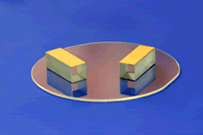CN

## Products

E-O Crystal
当前位置：
首页 >Products>Crystal>E-O Crystal
 When an electric field (E) is applied to an electro-optic (E-O) crystal, the refractive index of E-O crystal will change linearly to electric field. The phenomenon is called linear electro-optic effect. If a linearly polarized light passes through an E-O crystal, the phase retardation (Φ) will be induced by (ne-no) to Φ= 2p(ne-no)L, where L is crystal length, for KD*P, again as an example, Φ=pLno3r63E/l.  It is clear that the phase of light will change together with electric field (E). This is called electro-optic phase modulation.If two crossed polarizers are placed at input and output ends of E-O crystal separately, the output intensity of light will be I = I0sin2(Φ/2), where I0 is input intensity. That means the intensity or amplitude of light can also be modulated by electric field. This is called amplitude modulation.

There are two kinds of E-O modulations. One is longitudinal E-O modulation if the directions of electric field and light propagation are the same. The KDP isomorphic crystals are normally used in this scheme. If the directions of electric field and light propagation are perpendicular, it is called transverse E-O modulation. The LiNbO3, MgO:LiNbO3, ZnO:LiNbO3, BBO and KTP crystals are usually employed in this scheme.

The half-wave voltage (Vp) is defined as the voltage at Φ= l, for example, Vp=l/(2no3r63)  for KD*P and Vp=ld/(2no3r22L) for LiNbO3, where l is light wavelength and d is the distance between the electrodes.

BBO crystal is an excellent electro-optic crystal for high power applications at the wavelength range from 200nm to 2500nm. It has electro-optic coefficients g11=2.7pm/v, g22, g31<0.1g11. It can be used for Q-Switching a cw diode pumped Nd:YAG laser with average power>50W.

LiNbO3 is widely used as electro-optic modulator and Q-switch for Nd:YAG, Nd:YLF and Ti:Sapphire lasers as well as modulator for fiber optics, etc. If the light propagates in z-axis and electric field applies to x-axis, the refractive retardation will be G = pLnr22V/ld. The electro-optic coefficients of LiNbO3 are: r33 = 32 pm/V, r31 = 10 pm/V, r22 = 6.8 pm/V at low frequency and r33 = 31 pm/V, r31 = 8.6 pm/V, r22 = 3.4 pm/V at high electric frequency.

KTP has promising E-O and dielectric properties comparable to those of LiNbO3, which makes it extremely useful to various E-O devices. When these properties are combined with high damage threshold, low optical loss at high average power, wide optical bandwidth, thermal and mechanical stability, KTP crystals are expected to replace LiNbO3 crystals as E-O modulators, especially for mode-locking diode laser pumped Nd:YAG and Nd:YLF lasers as well as Ti:Sapphire and Cr:LiSrAlF6 lasers. KTP crystal was successfully used to mode lock a diode laser pumped Nd:YLF laser at 270MHz with pulse width of 15 ps and spectral band width of 73 GHz.

The following Table gives the comparison of KTP and those commonly used E-O modulator materials.

 Material Phase Amplitude e n r (pm/V) k (10-6/°C) n7r2/e(pm/V)2 r (pm/V) k (10-6/°C) n7r2/e(pm/V)2 KTP 15.4 1.80 35.0 31 6130 27.0 11.7 3650 LiNbO3 27.9 2.20 31.0 82 7410 20.1 42 3500 KD*P 48.0 1.47 26.4 9 178 24.0 8 178

KD*P crystal is widely applied for electro-optic application as Q-switch and Pockel cells.

 Crystal KDP KD*P ADP Electro-Optic Coefficient(pm/V) G63=10.5 G63=26.4 G41(T)=24.5 Longitudinal Half-Wave Voltage Vp(546nm) 7.65kV 2.98kV 9kV### 联系电话X

+86 27 87561503

+86 27 87734955